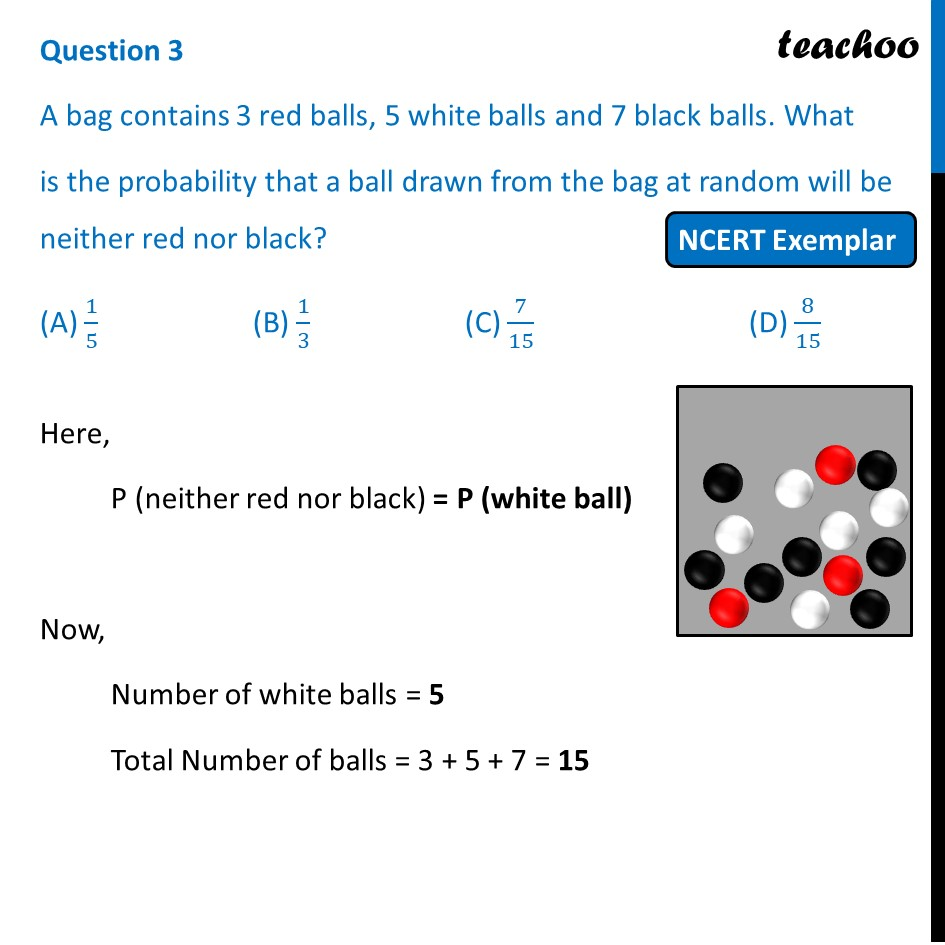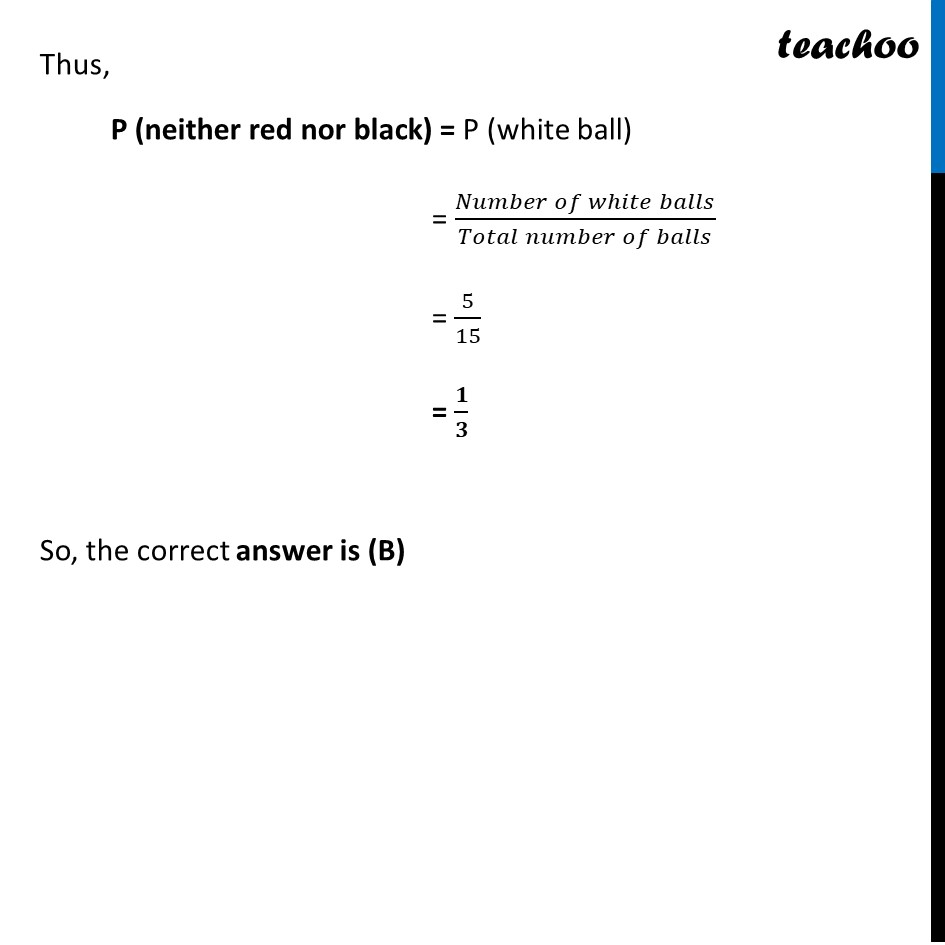NCERT Exemplar - MCQ

Chapter 14 Class 10 Probability
Serial order wise

## (A) 1/5   (B) 1/3   (C) 7/15   (D) 8/15Learn in your speed, with individual attention - Teachoo Maths 1-on-1 Class

### Transcript

Question 3 A bag contains 3 red balls, 5 white balls and 7 black balls. What is the probability that a ball drawn from the bag at random will be neither red nor black? (A) 1/5 (B) 1/3 (C) 7/15 (D) 8/15 Here, P (neither red nor black) = P (white ball) Now, Number of white balls = 5 Total Number of balls = 3 + 5 + 7 = 15 Thus, P (neither red nor black) = P (white ball) = (𝑁𝑢𝑚𝑏𝑒𝑟 𝑜𝑓 𝑤ℎ𝑖𝑡𝑒 𝑏𝑎𝑙𝑙𝑠)/(𝑇𝑜𝑡𝑎𝑙 𝑛𝑢𝑚𝑏𝑒𝑟 𝑜𝑓 𝑏𝑎𝑙𝑙𝑠) = 5/15 = 𝟏/𝟑 So, the correct answer is (B)